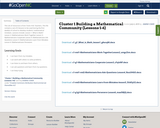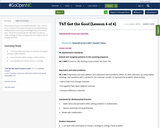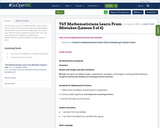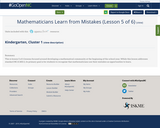Updating search results...

# 4 Results

View
Selected filters:
• mistakesConditional Remix & Share Permitted
CC BY-NC-SA
Rating
0.0 stars

This set of resources is from Tools 4 NC Teachers.

This file contains a set of 6 lessons to be used during the first week(s) of school to develop students' mathematical mindsets. Lessons include:
Lesson 1: What is Math?
Lesson 2: Mathematicians Work Together
Lesson 3: Mathematicians Cooperate
Lesson 5: Mathematicians Learn from Mistakes
Lesson 6: Mathematicians Persevere

Subject:
Mathematics
Material Type:
Lesson
Lesson Plan
11/06/2019Conditional Remix & Share Permitted
CC BY-NC-SA
Rating
0.0 stars

This resource is part of Tools4NCTeachers.

This is lesson six in a series of six lessons focused around developing a mathematical community at the beginning of the school year. The goal is to promote the idea that it is okay to make mistakes in math as long as we try to figure out the mistake and fix it.

Subject:
Mathematics
Material Type:
Lesson
Lesson Plan
11/06/2019Conditional Remix & Share Permitted
CC BY-NC-SA
Rating
0.0 stars

This resource is part of Tools4NCTeachers.

This is lesson five in a series of six lessons focused around developing a mathematical community at the beginning of the school year. While this lesson addresses standard NC.2.G.1, the primary goal is for students to recognize that mathematicians use their mistakes as opportunities to learn.

Subject:
Mathematics
Material Type:
Lesson
Lesson Plan
11/06/2019Conditional Remix & Share Permitted
CC BY-NC-SA
Rating
0.0 stars

This resource is part of Tools4NCTeachers.

This is lesson 5 of 6 lessons focused around developing a mathematical community at the beginning of the school year. While this lesson addresses standard NC.K.MD.3, its primary goal is for students to recognize that mathematicians use their mistakes as opportunities to learn.

Subject:
Mathematics
Material Type:
Lesson